# Experimental Investigation of the Evaporator Section Tilted Angle and Filling Ratio on the Thermal Charateristics of a Two-phase Closed Thermosyphon

Experimental Investigation of the Evaporator Section Tilted Angle and Filling Ratio on the Thermal Charateristics of a Two-phase Closed Thermosyphon
Department of Mechanical Engineering, Babol University of Technology, Babol 4714871167, Iran

Department of Chemical Engineering, University of Science and Technology of Mazandaran, Behshahr 4851878195, Iran

Corresponding Author Email:
m_r_emami@mazust.ac.ir
Page:
569-574
|
DOI:
https://doi.org/10.18280/ijht.370226
24 April 2018
|
Accepted:
10 June 2019
|
Published:
30 June 2019
| Citation

OPEN ACCESS

Abstract:

An experimental study was conducted in order to investigate the effect of filling ratio (F.R) and tilted angle (Φ) of the evaporator section on the thermal efficiency in a two-phase closed thermosyphon (TS) with the vertical condenser and adiabatic sections. Therefore, a copper tube with inner diameter of 14.5 mm was used with distilled water in the filling ratio 15 % to 60 % and input heat 100 to 250 watt. The evaporator section of TS (from the junction of an adiabatic section) is bent and compare with vertical positions. Through this study, it was found that optimum of F.R was 45 % and the best-tilted angle for the evaporator was 60º relative to the horizontal axis. Also, the boiling heat transfer coefficient rises about 15.2 % when the evaporator section will be bent  60º in optimized filling ratio. Furthermore, boiling heat transfer coefficient obtained from the experimental results of this research were compared with Rohsenow's correlation, who showed good agreement.

Keywords:

two-phase closed thermosyphon, tilted angle, filling ratio, Rohsenow's correlation, thermal performance

1. Introduction

The heat transfer is a section of engineering, and the always wishing energy efficiency provisions are driving engineers to continually improve the efficiency of heat transfer methods. The heat pipes are the best devices for heat transfer. The significant increase in heat pipe heat transfer, boiling and condensation of the two phenomena as well as the high-speed vapor of working fluid along the tube, is under vacuum conditions. The heat pipes without wicks, called two-phase closed thermosyphon (TS) [1-2]. As shown in Figure 1, TS, the working fluid within it, to get heat in the evaporator and then the loss of heat in the condenser, returns to the evaporator. The fluid in the TS, to return from the condenser to the evaporator, does not use a wick and do it with gravity. In this case, the evaporator should be lower than the position of the condenser in the direction of gravity ground .

TS performance is significantly affected by a large of parameters, such as geometry, the filling ratio of working fluid, properties of the working fluid, tilted angle, saturation conditions and etc. TS and other thermal devices for renewable energy applications such as in solar and geothermal can help you design more efficient, more cost-effective and greener systems . Also, it can use for the electronic cooling system , heat transfer in permafrost regions .

Booddachan et al. , investigated the effect of tilted angles, aspect ratios and Bond number on the thermal performance of a TS. They obtained the tilted angle with highest thermal performance ranged from 60º to 70º. Zuo et al.  studied the heat transfer of a tilted TS. They concluded that the minimum value of working fluid remains approximately constant from 20º to 90º, with relative to the horizontal, and by decreasing the tilted angle increase considerably. Terdtoon et al.  studied the effects of F.R., A.R (Aspect Ratio) and TS position angle, different working fluids and different diameters of TSs.

## 1.pngFigure 1. A schematic of a two-phased closed thermosyphon

TSs with tilted angle has better performance in the vertical position. It happens between 40º to 70º. Ong and Haider-E-Alahi  the effects of cooling flow rate and the filling ratio of R-134a on the thermal performance of a vertically tilted TS were also investigated experimentally. A.K. Mozumder et al  thermal performance of heat studied. Total heat transfer coefficient of the miniature heat pipe is found to be the higher for the Acetone as a pipe for various working fluids and filling ratios were working fluid. S.H. Noie et al.  were studied the effect of heat transfer rates, system pressure, aspect ratios and filling ratios on the heat transfer rate of a TS. The experimental results such as boiling and condensation heat transfer coefficients in TS were checked with the available correlations. M.R. Sarmasti Emami et al.  the effect of the aspect ratio and filling ratio on the thermal performance of a tilted TS has been studied experimentally. The best efficiency is in filling ratio of 45 % and at a tilted angle of 60º from horizontal. C.C. Hsu et al.  studied the effects of shock and vibration in the heat pipe with axis acceleration and tilted angle. They concluded that with increasing axis acceleration up to 1.5g total thermal resistance increases of 5 to 30 percent. Recently, researchers have focused on optimizing the properties of nanofluids for use in heat transfer devices i.e. heat exchangers and heat pipes [15-17]. Kamyar et al.  examined the effects of nanofluids on the performance of TS. Results indicate that both nanofluids improve the proficiency through a reduction in thermal resistance by 65 % (at 0.05 % volume for Al2O3) and 57 % (at 0.075 % volume for TiSiO4).

As previous studies have shown, the effect of tilted angle of TS and heat pipes was studied. In this research, the effect of tilted angle of evaporator section and filling ratio on the thermal characteristics of a TS was investigated. In Figure 2, the tilted angles of evaporator section are shown.

## 2.jpg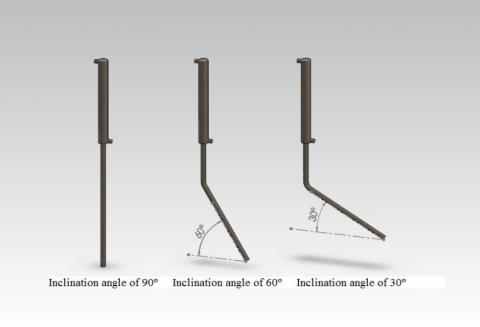Figure 2. Tilted angle of evaporator section

2. Exprimental Setup and Method

2.1 Experimental setup

In order to study the thermal efficiency of a two-phase closed thermosyphon, a specific experimental setup was designed and constructed. In this study, the tubes of copper were used with an internal diameter of 14.5 mm and the outer diameter of 16 mm. View a summary of the design specifications for the TS in Table 1.

Table 1. Design summary of the TS

 Internal Diameter (Di) 14.5 Outer Diameter (Do) 16 Adiabatic Length (La) 200 Condenser Length (Lc) 400 Evaporator Length (Le) 400 Aspect Ratio Le/Di (A.R) 28

Distilled water was used as the working fluid, because it has good thermophysical properties, i.e. The heat of vaporization is high and in the range of 25 to 100 °C can be used . Also, bending the evaporator section and the impact of changes the tilted angle on TS performance was evaluated. In this work the operating parameters studied were shown in Table 2.

Table 2. Operating parameter in this research

 TS angle (degree) Input power (watt) Filling ratio 90 100, 150, 200, 250 15%< F.R <60% 60 200 15%< F.R <60% 30 200 15%< F.R <60%

The thermal characteristics of the TS were accomplished by distributing the temperature on the outer surface of the tube.Thermocouples were used by RTD PT100). The accuracy of thermocouples is ±0.1 Celsius.

To control the input power to the tubes of a rheostat device with a nominal power of 1000W was used. Performance pressure determined with a pressure gauge that was connected to the top of the TS. The cooling water flow rate of a flow meter (Rotameter) was measured. As the rate of water entering the condenser was set to the temperature difference between the inlet and outlet reach to a level that was recognizable. Accordingly, the water flow rate of 250 (cm3/min) was determined. The thermosyphon was vacuumed down to 10 (psi). Input power can also be adjusted so that 1000 (W/m2) the heat flux was input to each TS. The tube with different diameters with a ratio of 15 %, 30 %, 45% and 60 % was tested. A schematic of the experimental setup is shown in Figure 3.

## 3.pngFigure 3. A schematic of the experimental setup

2.2 Uncertainty analysis

For the calculation of the uncertainty in the measured values of the tests using the manufacturer’s specification for each device on the Table 3.

Table 3. Uncertainty in measured parameters

 Relative Uncertainty Device Measurement ± 0.5 ºC RTD(PT100) thermocouple Temperature ± 5 ml Flow meter (Rotameter) Cooling water flow ± 3.2% Best DT9205A multimeter Voltage ± 2.2% Best DT9205A multimeter Current ± 3.8% Best DT9205A multimeter Electric resistance

By calculating the relative uncertainty of measurement values in Table 2 and putting in Eq. (1) was presented by Kline and McClintock  tests measure the amount of uncertainty is calculated:

$\Delta f=\sqrt{\sum_{i=1}^{i=n}\left(\frac{\partial f}{\partial x_{i}}\right) \Delta x_{i}}$  (1)

Consequently, the maximum value of uncertainty is for heat transfer coefficient about 4.06 %, the rate of output heat transfer about 3.2 %, input power about 3.8 %, and efficiency about 4.9 %.

2.3 Heat transfer rate

Input power and output heat, the most important value will be calculated on the basis of the results obtained. Using the following equation can be input voltage and electrical current, the actual power rate input in the evaporator section can be calculated by Eq. (2):

$\dot{Q}_{i n}=V . I-\dot{Q}_{l o s s}$  (2)

The rate of dissipated heat, as well as the following equation of heat dissipation through convection and radiation, can be obtained from Eq. (3):

$\dot{Q}_{l o s s}=\dot{Q}_{r a d .}+\dot{Q}_{c o n v .}$  (3)

The rate of heat dissipated through radiation and convection around the tube insulation is calculated as Eqns. (4) and (5):

$\dot{Q}_{r a d .}=\varepsilon . \sigma . A_{e}\left(T_{i n s .}^{4}-T_{a i r}^{4}\right)$  (4)

$\dot{Q}_{c o n v .}=h_{c o n v .} A_{e}\left(T_{i n s .}-T_{a i r}\right)$  (5)

Heat transfer coefficient around the vertical and tilted cylinder is calculated from the relationship between Churchill and Chu by Eq. (6), where Ra (Rayleigh number) of Eq. (7) is obtained:

$N u=\frac{h_{c o n v .} L_{e}}{k_{a i r}}=\left\{0.825+\frac{0387 R a^{1 / 6}}{\left[1+\left(\frac{0492}{\mathrm{Pr}}\right)^{9 / 16}\right]^{8 / 27}}\right\}^{2}$           (6)

$R a=\frac{g \beta\left(T_{i n s}-T_{a i r}\right) L_{e}^{3}}{\alpha v}$      （7）

Incropera and De Witt noted that for 30 < Φ <90, g can be replaced by gsinΦ in Eq. (7) can be used .

Heat transfer rate output from the Eq. (8) based on the difference in temperature around the condenser cooling water is obtained:

$\dot{Q}_{o u t}=\dot{m} c_{p, w}\left(T_{w, o u t}-T_{w, i n}\right)$      （8）

2.4 Evaporator heat transfer coefficient

Experimental evaporation heat transfer coefficient was evaluated from the Eq. (9).

$h_{e}=\frac{\dot{Q}_{o u t}}{A_{e}\left(T_{v}-T_{e v a}\right)}$      （9）

where Teva., The average temperature of evaporator surface and Tv is vapor temperature that, in this research, was considered the adiabatic surface temperature based on older studies [22-23].

It was found that nucleate boiling is the predominant mechanism in the evaporator section. Therefore, a correlation based on nucleate boiling were selected to compare with the experimental data obtained from this research. Rohsenow  correlated experimental data for nucleate pool boiling with Eq. (10):

$h_{e}=\frac{q_{e}^{2 / 3}}{\frac{c_{s f} h_{f g}}{c_{p}}}\left\{\frac{1}{h_{f g} \mu}\left(\frac{\sigma}{g\left(\rho_{l}-\rho_{v}\right)}\right)^{1 / 2}\right\}^{0.33} \operatorname{Pr}$           （10）

The values of the Csf are given in reference .

3. Results and Discussion

A series of experiments were carried out to find the effect of varying parameters on the thermal characteristics of the two-phase closed thermosyphon.

3.1 The temperature distribution

The Figures 4 and 5 show the variation of the temperature along the length of the TS in the various tilted angles of evaporator section.

## 4.pngFigure 4. Variation of the temperature along the Ts with the Φ=90º (vertical evaporator)

## 5.pngFigure 5. Variation of the temperature along the TS with the evaporator with Φ=60º

As can be seen in the above Figures 4 and 5, the evaporator surface temperature in the approximate shape remains constant. But in the adiabatic section, the temperature is reduced suddenly because of the input heat flux was not in this, the temperature difference between the evaporator and condenser is minimum in filling ratio of 45 %. Therefore the total thermal resistance is lowest in the pipe. The performance of the TS will be better at this filling ratio. Also, the reason for the rising temperature in the evaporator section at filling ratio of 15 % can local drying of this section.

3.2 Effect of filling ratio

Change the amount of fluid in the TS is one of the very important factors affecting the performance of TS. Therefore, The filling ratio of fluid 15 %, 30 %, 45 % and 60 % were studied. Figure 6 shows the variation of efficiency (Qout/Qin), by changing the filling ratio in the various tilted angles of the evaporator.

Which shows the highest efficiency for filling ratio will be off 45 % and tilted angle of 60º. With an increasing filling ratio, due to the increased intensity of geyser boiling, efficiency is reduced. However, to increase the tilted angle to 60º decreases the effect of this phenomenon .

## 6.png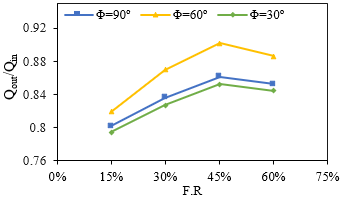Figure 6. Variation of Qout/Qin with the filling ratio for three tilted angles of evaporator

## 7.pngFigure 7. Variation of Qout/Qin with the filling ratio for all input power for Φ=90º

Figure 7 shows the variation of the thermal efficiency (Qout/Qin), respect to the filling ratio of the various input power.

It can be seen that the maximum thermal efficiency takes place in the F.R=45 % and Qin =250W for Φ=90°. Also by increasing the filling ratio to 60 % in Qin=250W, the thermal efficiency of TS decreased. Because input power of 250W is higher than the value of the boiling limits for this filling ratio and TS geometry .

3.3 Effect of tilted angle of evaporator

With bending the pipe of the evaporator and adiabatic junction, will be considered the tilted angle of the evaporator. Therefore, the effect of the tilted angle of the evaporator section at 90º, 60º and 30° relative to the horizontal axis was investigated. The thermal efficiency for tilted angle relative to vertical TS in different F.R is shown in Figure 8.

## 8.png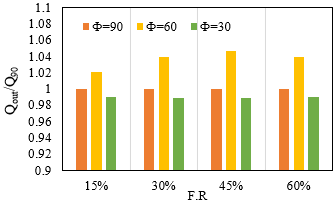Figure 8. Variation of Qout/Q90 with the tilted angle

As shown in Figure 8, the maximum thermal efficiency of TS occurs at 60º of a tilted angle and an optimum filling ratio (F.R.=45 %).

Figure 9 shows that the evaporator heat transfer coefficient increased when the tilted angle of evaporator changed from vertical to 60˚ and decreased when it bent to 30˚.

## 9.png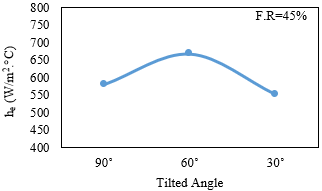Figure 9. Variation of he with the tilted angle for F.R=45 %

## 10.png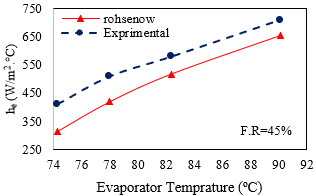Figure 10. Comparison of boiling heat transfer coefficient with Rohsenow correlation  for F.R=45 %

As explained earlier, the experimental results of this research can be compared with Rohsenow correlation in Eq. (10). As shown in Figure 10, a good agreement (around 15 % on average) was observed between the results obtained from this study with Rohsenow correlation in the F.R.=45%.

4. Conclusions

In this research, the thermal characteristics of a two-phase closed thermosyphon (TS) with distilled water were studied experimentally. This investigation is mainly concerned with the effect of the evaporator section tilted angle at various filling ratio and input heat. The main results were as follows:

*  The maximum thermal efficiency of TS occurs at F.R=45 % and Φ=60º of evaporator section.

*  The maximum output heat transfer rate for all filling ratios occurs at 60º.

*  The geyser boiling phenomenon happened during the experiments for filling ratios greater than 45%. Therefore, in this situation, the thermal efficiency of TS was reduced more quickly.

*  The average temperature of the evaporator surface declined in optimum filling ratio and represents minimum total thermal resistance.

*  Increasing the input heat will be caused by the increased the thermal efficiency, as long as it does not pass the boiling limited heat transfer.

*  Application of various nanofluid as a working fluid and different geometry (i.e. length and diameter) of TS are recommendations for future studies in this situation.

Nomenclature

Ae         evaporator lateral surface, m2

A.R       aspect ratio

cp,w     specific heat of water, J.kg-1.°C-1

Csf       constant, determined from experimental data

Di          internal diameter of pipe, m

Do         outlet diameter of pipe, m

F.R         filling ratio (=Vl/Ve)

g            gravitational acceleration, m.s-2

hcov.      convection heat transfer coefficient, W.m-2.°C-1

he          heat transfer coefficient at evaporator, W.m-2.°C-1

hfg         latent heat of vaporization, J.kg-1

I              current, A

kair         thermal conductivity of air, W.m-1.°C-1

kl            thermal conductivity of liquid, W.m-.°C-1

Lc           condenser length, m

Le           evaporator length, m

$\dot{m}$           mass flow rate of water, kg.s-1

Nu           Nusselt number (=hconv. Le / kair)

Pr            Prandtl number (= υ/α)

qe            input heat flux, W.m-2

Qin          input heat into the evaporator section, W

Qcov        convection heat transfer rate, W

Qloss       heat loss by radiation and convection, W

Qout        transmitted heat from the condenser section, W

Patm        standard atmospheric pressure, Pa

Psat         vapor saturation pressure, Pa

Ra           Rayleigh number (= $g \beta\left(T_{i n s}-T_{a i r}\right) L_{e}^{3} / \alpha v$)

Tair         air temperature, °C

Teva        average temperature of evaporator section, °C

Tins         temperature on external surface of insulation, °C

Tw.in        inlet water temperature of condenser, °C

Tw.o        outlet water temperature of condenser, °C

Tv            vapor temperature, °C

V             voltage, V

Ve           evaporator volume, m3

Vl            liquid volume, m3

Greek symbols

α           thermal diffusivity of air, m2.s-1, (=k/ ρ.cp)

β           inverse of film temperature of air, K-1, (=$\frac{1}{T_{f}}$)

ν           kinematic viscosity of air, m2.s-1, (=μ/ ρ)

l            viscosity of liquid, kg.m-1s-1

ρl          density of liquid, kg.m-3

ρv         density of vapor, kg.m-3

σ           Stefan Boltzmann constant in Eq.(4) (W/m2.K4)

ε           emissive factor of insulator

Φ          tilted angle (measured against horizontal)

References

 Faghri, A. (2012). Review and advances in heat pipe science and technology. Journal of Heat Transfer, 134(12): 123001-123018. http://doi.org/10.1115/1.4007407

 Noie, S.H., Sarmasti Emami, M.R., Khoshnoodi, M. (2007). Effect of inclination angle on thermal performance of a two-phase closed thermosyphon under normal operating condition. Heat Transf. Eng. 28(4): 365-371. https://doi.org/10.1080/01457630601122997

 Peterson, G.P. (1994). An introduction to Heat Pipe Modeling, Testing and Applications. John Wiley & Sons, Hoboken, USA.

 Jafari, D., Franco, A., Filippeschi, S., Di Marco, P. (2016). Two-phase closed thermosyphons: A review of studies and solar applications. Renewable & Sustainable Energy Reviews, 53: 575-593. http://doi.org/10.1016/j.rser.2015.09.002

 Gopi Kannan, K., Kamatchi, R., Venkatajalapathi, T., Krishnan, A.S. (2018). Enhanced heat transfer by thermosyphon method in electronic devices. Int. J. of Heat and Technology, 36(1): 339-343. https://doi.org/10.18280/ijht.360145

 Zhang, M., Lai, Y., Zhang, J., Sun, Z. (2011). Numerical study on cooling characteristics of two-phase closed thermosyphon embankment in permafrost regions. Cold Regions Science and Technology, 65(2): 203-210. https://doi.org/10.1016/j.coldregions.2010.08.001

 Booddacham, K., Tantakom, P., Terdtoon, P., Polchai, A. (1996). Thermal behavior of a HPDE thermosyphon. Proc. of the 5th Int. Heat Pipe Symposium, Melbourne, Australia.

 Zuo, Z.J., Gunnerson, F.S. (1995). Heat transfer analysis of an tilted two-phase thermosyphon. Journal of Heat Transfer, 117: 1073-1075. http://doi.org/10.1115/1.2836287

 Terdtoon, P., Ritthidech, S., Shiraishi, M. (1996). Effect of aspect ratio and bond number on heat transfer characteristics of an tilted two-phase closed thermosyphon at normal operating condition. Proceeding of the 5th Int. Heat Pipe Symp, Melbourne, Australia, pp. 261-266.

 Ong, K.S., Haider-E-Alahi, M. (2003). Performance of a R-134a filled thermosyphon. Applied Thermal Engineering, 23(18): 2373-2381. http://doi.org/10.1016/s1359-4311(03)00207-2

 Mozumder, A.K., Akon, A.F., Chowdhury M.S.H., Banik, S.C. (2010). Performance of heat pipe for different working fluids and fill ratios. J. of Mechanical Engineering, 41(2): 96-102. http://dx.doi.org/10.3329/jme.v41i2.7473

 Noie, S.H., Kalaei, M.H., Khoshnoodi, M. (2005). Exprimental investigation of boiling and condensation heat transfer of a two phase closed thermosyphon. Int. J. of Eng. 18(1): 37-43.

 Sarmasti Emami, M.R., Noie, S.H., Khoshnoodi, M. (2008). Effect of aspect ratio and filling ratio on thermal performance of an tilted two-phase closed thermosyphon. Iranian Journal of Science and Technology Transaction B: Engineering, 32(B1): 39-51.

 Hsu, C.C., Chen, X.F., Yang, J.M. (2011). The effects of shock and vibration on heat pipe performance in reliability tests. 10th Int. Heat Pipe Symp., Taipei, Taiwan, pp. 6-9.

 Esfe, H.M., Rostamian, H., Rejvani, M., Sarmasti Emami, M.R. (2018). Rheological behavior characteristics of ZrO2-MWCNT/10w40 hybrid nano-lubricant affected by temperature, concentration, and shear rate: An experimental study and a neural network simulating. Physica E: Low-dimensional Systems and Nanostructures, 102: 160-170. https://doi.org/10.1016/j.physe.2017.12.023

 Hemmat Esfe, M., Rahimi Raki, H., Sarmasti Emami, M.R., Afrand, M. (2019). Viscosity and rheological properties of antifreeze based nanofluid containing hybrid nano-powders of MWCNTs and TiO2 under different temperature conditions. Powder Technology, 342: 808-816. https://doi.org/10.1016/j.powtec.2018.10.032

 Hemmat Esfe, M., Sarmasti Emami, M.R., Kiannejad Amiri, M. (2019). Experimental investigation of effective parameters on MWCNT–TiO2/SAE50 hybrid nanofluid viscosity. Journal of Thermal Analysis and Calorimetry, in Press. https://doi.org/10.1007/s10973-018-7986-0

 Kamyar, A., Ong, K.S., Saidur, R. (2013). Effects of nanofluids on heat transfer characteristics of a two-phase closed thermosyphon. International Journal of Heat and Mass Transfer, 65: 610–618. https://doi.org/10.1016/j.ijheatmasstransfer.2013.06.046

 Kline, S.J., McClintock, F.A. (1953). Describing uncertainties in single-sample experiments. Mech. Eng. 75(1): 3-8.

 Churchill, S.W., Chu, H.H.S. (1975). Correlating equations for laminar and turbulent free convection from a vertical plate. International Journal of Heat and Mass Transfer, 18(11): 1323-1329. https://doi.org/10.1016/0017-9310(75)90243-4

 Incropera, F.P., DeWitt, D.P. (2002). Introduction to Heat Transfer. 4th Edition, John Wiley & Sons Australia, Chap.9.

 Huber, N., Bowman, W. (1996). Longitudinal vibration effects on a copper/water heat pipe’s capillary limits. Journal of Thermophysics and Heat Transfer, 10(1): 90-96. http://arc.aiaa.org/doi/10.2514/3.757

 Abou-Ziyan, H.Z., Helali, A., Fatouh, M., Abo El-Nasr, M.M. (2001). Performance of stationary and vibrated thermosyphon working with water and R134a. Applied Thermal Engineering, 21: 813-830. https://doi.org/10.1016/S1359-4311(00)00089-2

 Rohsenow, W.M. (1962). A method of correlating heat transfer data for surface boiling curves. Int. J. of Heat Transfer ASME, 84: 969-976. http://hdl.handle.net/1721.1/61431

 Pioro, I. (1998). Experimental evaluation of constants for the Rohsenow pool boiling correlation. International Journal of Heat and Mass Transfer, 42(11): 2003-2013. http://doi.org//10.1016/S0017-9310(98)00294-4

 Sarmasti Emami, M.R., Noie, S.H., Khoshnoodi, M., Hamed Mosavian, M.T., Kianifar, A. (2009). Investigation of geyser boiling phenomenon in a two-phase closed thermosyphon. Heat Transfer Engineering, 30(5): 408-415. https://doi.org/10.1080/014576302414979